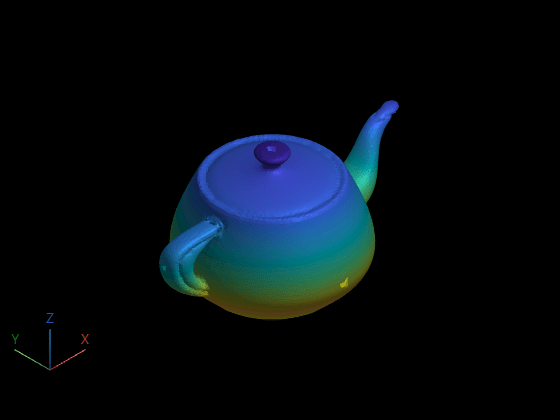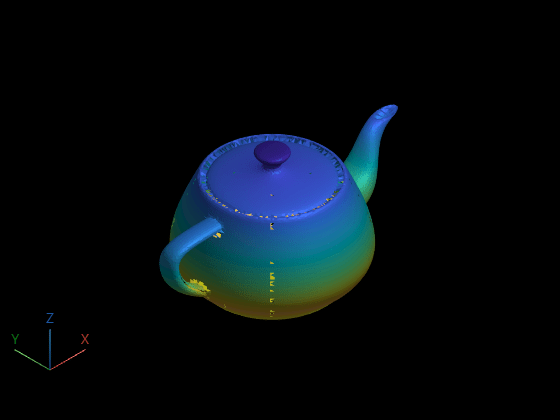# pc2surfacemesh

Construct surface mesh from 3-D point cloud

Since R2022b

## Syntax

``[mesh,depth,perVertexDensity] = pc2surfacemesh(ptCloudIn,"poisson")``
``[mesh,depth,perVertexDensity] = pc2surfacemesh(ptCloudIn,'poisson',inputDepth)``
``[mesh,radii] = pc2surfacemesh(ptCloudIn,"ball-pivot")``
``[mesh,radii] = pc2surfacemesh(ptCloudIn,'ball-pivot',inputRadii)``

## Description

example

````[mesh,depth,perVertexDensity] = pc2surfacemesh(ptCloudIn,"poisson")` creates a surface mesh from the input point cloud `ptCloudIn` using the Poisson reconstruction method. The function also returns the octree depth used in the reconstruction `depth` and the vertex density `perVertexDensity`. ```
````[mesh,depth,perVertexDensity] = pc2surfacemesh(ptCloudIn,'poisson',inputDepth)` additionally specifies the octree depth value for the Poisson reconstruction method.```

example

````[mesh,radii] = pc2surfacemesh(ptCloudIn,"ball-pivot")` constructs a surface mesh from point cloud data using the ball-pivot method. The function also returns the radii used in the reconstruction.```
````[mesh,radii] = pc2surfacemesh(ptCloudIn,'ball-pivot',inputRadii)` additionally specifies the radii for the ball-pivot reconstruction method.```

## Examples

collapse all

Load point cloud data from a PLY file into the workspace.

`ptCloud = pcread("teapot.ply");`

Display the input point cloud.

`pcshow(ptCloud)`Downsample the point cloud.

```gridstep = 0.05; ptCloudDownSampled = pcdownsample(ptCloud,"gridAverage",gridstep);```

Construct surface mesh from the point cloud data using the Poisson method, and display the surface mesh.

```depth = 8; mesh = pc2surfacemesh(ptCloudDownSampled,"poisson",depth); surfaceMeshShow(mesh)```Load point cloud data from a PLY file into the workspace.

`ptCloud = pcread("teapot.ply");`

Display the input point cloud.

`pcshow(ptCloud)`Downsample the point cloud.

```gridstep = 0.05; ptCloudDownSampled = pcdownsample(ptCloud,"gridAverage",gridstep);```

Construct a surface mesh from the point cloud data using the ball-pivot method and display the surface mesh.

```mesh = pc2surfacemesh(ptCloudDownSampled,"ball-pivot"); surfaceMeshShow(mesh)```## Input Arguments

collapse all

Input point cloud data, specified as a `pointCloud` object.

Octree depth to use in Poisson reconstruction, specified as a positive integer in the range `[2, 12]`. Increasing the octree depth of the Poisson reconstruction increase the detail of the surface mesh.

Data Types: `single` | `double` | `int8` | `int16` | `int32` | `int64` | `uint8` | `uint16` | `uint32` | `uint64`

Radii values for ball-pivot reconstruction, specified as an M-element vector. You must specify the values depending on the point cloud density. Values are in meters.

Data Types: `single` | `double` | `int8` | `int16` | `int32` | `int64` | `uint8` | `uint16` | `uint32` | `uint64`

## Output Arguments

collapse all

Surface mesh constructed from the point cloud, returned as a `surfaceMesh` object.

Octree depth used in the Poisson reconstruction, returned as a positive integer.

Radii values for the ball-pivot reconstruction, returned as a three-element vector. Units are in meters.

Density at mesh vertices, returned as an M-element vector. M is the number of mesh vertices in the output surface mesh. You can further refine the mesh by eliminating vertices with insignificant density.

## Algorithms

collapse all

### Poisson Reconstruction

The Poisson reconstruction method consists of these steps.

1. Transform the point samples into a continuous vector field.

2. Solve a Poisson system, containing 3-D Laplacian equations, to find a function whose gradient best describes the point cloud.

3. Reconstruct the surface from the function equation.

### Ball-Pivot Reconstruction

The ball-pivot method triangulates a set of points by rolling a ball, of radius r, on the point cloud. The algorithm consists of these steps.

1. Place the ball in contact with three sample points. These points form the seed triangle.

2. Keep the ball in contact with two of these initial points (an edge of the seed triangle) and pivot the ball until it touches another point. The edge and the new point define a new triangle.

3. Pivot the ball using the new triangle edge. Using an edge of the new triangle, repeat the process of pivoting and defining a new triangle with a touched point. The triangles formed through this process constitute the interpolating mesh.

4. Continue this process until all reachable edges are covered, and then start with another seed triangle.

5. Repeat the entire process with larger radii to reconstruct uneven surfaces.

## Version History

Introduced in R2022b

expand all